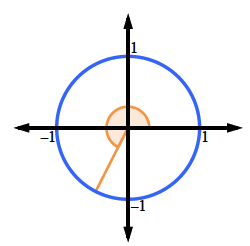Home > A2C > Chapter 8 > Lesson 8.1.6 > Problem8-91

8-91.
1. Calculate the value of each expression below. Each measure is given in radians. Homework Help ✎

1. sin(4)

2.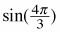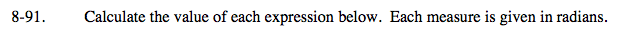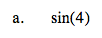Make sure your calculator is in radian mode.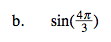$\text{Since }\frac{4\pi}{3} \text{ is related to the }30\degree-60\degree-90\degree\text{ angles, this one}$

$\text{has an exact answer. Locate it on the unit circle.}$

$\text{Notice that it will be a reflection (twice) of sin} \left(\frac{\pi}{3}\right).$

It is in the third quadrant.

−0.76